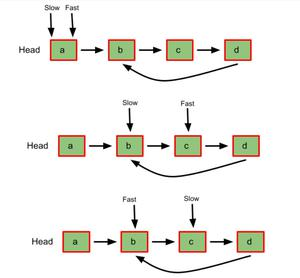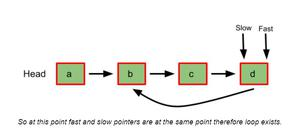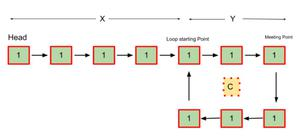Open In App

# Floyd’s Cycle Finding Algorithm

Floyd’s cycle finding algorithm or Hare-Tortoise algorithm is a pointer algorithm that uses only two pointers, moving through the sequence at different speeds. This algorithm is used to find a loop in a linked list. It uses two pointers one moving twice as fast as the other one. The faster one is called the fast pointer and the other one is called the slow pointer.

How Does Floyd’s Cycle Finding Algorithm Works?

While traversing the linked list one of these things will occur-

• The Fast pointer may reach the end (NULL) this shows that there is no loop in the linked list.
• The Fast pointer again catches the slow pointer at some time therefore a loop exists in the linked list.

Example:Pseudocode:

• Initialize two-pointers and start traversing the linked list.
• Move the slow pointer by one position.
• Move the fast pointer by two positions.
• If both pointers meet at some point then a loop exists and if the fast pointer meets the end position then no loop exists.

Below is the C++ program to implement the above approach:

## C++

 `// C++ program to implement``// the above approach``#include ``using` `namespace` `std;` `class` `Node {``public``:``    ``int` `data;``    ``Node* next;` `    ``Node(``int` `data)``    ``{``        ``this``->data = data;``        ``next = NULL;``    ``}``};` `// initialize a new head for the linked list``Node* head = NULL;``class` `Linkedlist {``public``:``    ``// insert new value at the start``    ``void` `insert(``int` `value)``    ``{``        ``Node* newNode = ``new` `Node(value);``        ``if` `(head == NULL)``            ``head = newNode;``        ``else` `{``            ``newNode->next = head;``            ``head = newNode;``        ``}``    ``}` `    ``// detect if there is a loop``    ``// in the linked list``    ``bool` `detectLoop()``    ``{``        ``Node *slowPointer = head,``             ``*fastPointer = head;` `        ``while` `(slowPointer != NULL``               ``&& fastPointer != NULL``               ``&& fastPointer->next != NULL) {``            ``slowPointer = slowPointer->next;``            ``fastPointer = fastPointer->next->next;``            ``if` `(slowPointer == fastPointer)``                ``return` `1;``        ``}` `        ``return` `0;``    ``}``};` `int` `main()``{``    ``Linkedlist l1;``    ``// inserting new values``    ``l1.insert(10);``    ``l1.insert(20);``    ``l1.insert(30);``    ``l1.insert(40);``    ``l1.insert(50);` `    ``// adding a loop for the sake``    ``// of this example``    ``Node* temp = head;``    ``while` `(temp->next != NULL)``        ``temp = temp->next;` `    ``temp->next = head;` `    ``// loop added;` `    ``if` `(l1.detectLoop())``        ``cout << ``"Loop exists in the"``             ``<< ``" Linked List"` `<< endl;``    ``else``        ``cout << ``"Loop does not exists "``             ``<< ``"in the Linked List"` `<< endl;` `    ``return` `0;``}`

## Java

 `// Java program to implement``// the above approach` `import` `java.util.*;` `class` `GFG{` `static` `class` `Node {``    ``int` `data;``    ``Node next;` `    ``Node(``int` `data)``    ``{``        ``this``.data = data;``        ``next = ``null``;``    ``}``};` `// initialize a new head for the linked list``static` `Node head = ``null``;``static` `class` `Linkedlist {``    ``// insert new value at the start``    ``void` `insert(``int` `value)``    ``{``        ``Node newNode = ``new` `Node(value);``        ``if` `(head == ``null``)``            ``head = newNode;``        ``else` `{``            ``newNode.next = head;``            ``head = newNode;``        ``}``    ``}` `    ``// detect if there is a loop``    ``// in the linked list``    ``boolean` `detectLoop()``    ``{``        ``Node slowPointer = head,``             ``fastPointer = head;` `        ``while` `(slowPointer != ``null``               ``&& fastPointer != ``null``               ``&& fastPointer.next != ``null``) {``            ``slowPointer = slowPointer.next;``            ``fastPointer = fastPointer.next.next;``            ``if` `(slowPointer == fastPointer)``                ``return` `true``;``        ``}` `    ``return` `false``;``    ``}``}` `public` `static` `void` `main(String[] args)``{``    ``Linkedlist l1 = ``new` `Linkedlist();``    ``// inserting new values``    ``l1.insert(``10``);``    ``l1.insert(``20``);``    ``l1.insert(``30``);``    ``l1.insert(``40``);``    ``l1.insert(``50``);` `    ``// adding a loop for the sake``    ``// of this example``    ``Node temp = head;``    ``while` `(temp.next != ``null``)``        ``temp = temp.next;` `    ``temp.next = head;` `    ``// loop added;` `    ``if` `(l1.detectLoop())``        ``System.out.print(``"Loop exists in the"``            ``+ ``" Linked List"` `+``"\n"``);``    ``else``        ``System.out.print(``"Loop does not exists "``            ``+ ``"in the Linked List"` `+``"\n"``);` `}``}` `// This code is contributed by 29AjayKumar`

## Python3

 `# Python code for the above approach``class` `Node:``    ``def` `__init__(``self``, d):``        ``self``.data ``=` `d``        ``self``.``next` `=` `None` `# initialize a new head for the linked list``head ``=` `None` `# detect if there is a loop``# in the linked list``def` `detectLoop(head):``    ``slowPointer ``=` `head``    ``fastPointer ``=` `head` `    ``while` `(slowPointer !``=` `None``           ``and` `fastPointer !``=` `None``           ``and` `fastPointer.``next` `!``=` `None``):``        ``slowPointer ``=` `slowPointer.``next``        ``fastPointer ``=` `fastPointer.``next``.``next``        ``if` `(slowPointer ``=``=` `fastPointer):``            ``return` `1` `    ``return` `0` `# inserting new values``head ``=` `Node(``10``)``head.``next` `=` `Node(``20``)``head.``next``.``next` `=` `Node(``30``)``head.``next``.``next``.``next` `=` `Node(``40``)``head.``next``.``next``.``next``.``next` `=` `Node(``50``)` `# adding a loop for the sake``# of this example``temp ``=` `head``while` `(temp.``next` `!``=` `None``):``    ``temp ``=` `temp.``next` `temp.``next` `=` `head` `# loop added;``if` `(detectLoop(head)):``    ``print``(``"Loop exists in the Linked List"``)``else``:``    ``print``(``"Loop does not exists in the Linked List"``)` `# This code is contributed by Saurabh Jaiswal`

## C#

 `// C# program to implement``// the above approach``using` `System;``using` `System.Collections.Generic;``public` `class` `GFG {` `  ``public` `class` `Node {``    ``public` `int` `data;``    ``public` `Node next;` `    ``public` `Node(``int` `data) {``      ``this``.data = data;``      ``next = ``null``;``    ``}``  ``};` `  ``// initialize a new head for the linked list``  ``static` `Node head = ``null``;` `  ``public` `class` `Linkedlist``  ``{` `    ``// insert new value at the start``    ``public` `void` `insert(``int` `value) {``      ``Node newNode = ``new` `Node(value);``      ``if` `(head == ``null``)``        ``head = newNode;``      ``else` `{``        ``newNode.next = head;``        ``head = newNode;``      ``}``    ``}` `    ``// detect if there is a loop``    ``// in the linked list``    ``public` `bool` `detectLoop() {``      ``Node slowPointer = head, fastPointer = head;` `      ``while` `(slowPointer != ``null` `&& fastPointer != ``null` `&&``             ``fastPointer.next != ``null``)``      ``{``        ``slowPointer = slowPointer.next;``        ``fastPointer = fastPointer.next.next;``        ``if` `(slowPointer == fastPointer)``          ``return` `true``;``      ``}` `      ``return` `false``;``    ``}``  ``}` `  ``public` `static` `void` `Main(String[] args) {``    ``Linkedlist l1 = ``new` `Linkedlist();``    ``// inserting new values``    ``l1.insert(10);``    ``l1.insert(20);``    ``l1.insert(30);``    ``l1.insert(40);``    ``l1.insert(50);` `    ``// adding a loop for the sake``    ``// of this example``    ``Node temp = head;``    ``while` `(temp.next != ``null``)``      ``temp = temp.next;` `    ``temp.next = head;` `    ``// loop added;``    ``if` `(l1.detectLoop())``      ``Console.Write(``"Loop exists in the"` `+ ``" Linked List"` `+ ``"\n"``);``    ``else``      ``Console.Write(``"Loop does not exists "` `+ ``"in the Linked List"` `+ ``"\n"``);` `  ``}``}` `// This code is contributed by umadevi9616`

## Javascript

 ``

Output

```Loop exists in the Linked List
```

Time complexity: O(n), as the loop is traversed once.
Auxiliary Space: O(1), only two pointers are used therefore constant space complexity.

Why Does Floyd’s Algorithm Works?

Let us consider an example:• Let,

X = Distance between the head(starting) to the loop starting point.

Y = Distance between the loop starting point and the first meeting point of both the pointers.

C = The distance of the loop

• So before both the pointer meets-

The slow pointer has traveled X + Y + s * C distance, where s is any positive constant number.

The fast pointer has traveled X + Y + f * C distance, where f is any positive constant number.

• Since the fast pointer is moving twice as fast as the slow pointer, we can say that the fast pointer covered twice the distance the slow pointer covered. Therefore-

X + Y + f * C = 2 * (X + Y + s * C)

X + Y = f * C – 2 * s * C

We can say that,

f * C – 2 * s * C = (some integer) * C

= K * C

Thus,

X + Y = K * C       – ( 1 )

X = K * C – Y        – ( 2 )

Where K is some positive constant.

• Now if reset the slow pointer to the head(starting position) and move both fast and slow pointer by one unit at a time, one can observe from 1st and 2nd equation that both of them will meet after traveling X distance at the starting of the loop because after resetting the slow pointer and moving it X distance, at the same time from loop meeting point the fast pointer will also travel K * C – Y distance(because it already has traveled Y distance).
• From equation (2) one can say that X = K * C – Y therefore, both the pointers will travel the distance X i.e. same distance after the pink node at some point to meet at the starting point of the cycle.
• Here, by some point, it means that the fast pointer can complete the K * C distance out of which it has already covered the Y distance.
• Conclusively, we find that slow pointer moves 1 step at a time while fast pointer moves 2 steps at a time so the fast pointer catches or surpasses slow pointer if there is a loop, just like start <end in Binary Search. Else if there exists no loop then fast pointer reaches the NULL before than slow (and wins the race: tortoise ->slow pointer and hare/rabbit->fast pointer).

Below is the C++ program to implement the above approach-

## C++

 `// C++ program to implement``// the above approach``#include ``using` `namespace` `std;` `class` `Node {``public``:``    ``int` `data;``    ``Node* next;` `    ``Node(``int` `data)``    ``{``        ``this``->data = data;``        ``next = NULL;``    ``}``};` `// initialize a new head``// for the linked list``Node* head = NULL;``class` `Linkedlist {``public``:``    ``// insert new value at the start``    ``void` `insert(``int` `value)``    ``{``        ``Node* newNode = ``new` `Node(value);``        ``if` `(head == NULL)``            ``head = newNode;``        ``else` `{``            ``newNode->next = head;``            ``head = newNode;``        ``}``    ``}` `    ``// detect if there is a loop``    ``// in the linked list``    ``Node* detectLoop()``    ``{``        ``//If link list is empty, there are no loop.``        ``//If only one element is found in link list, there are no loop.``        ``if``(head==NULL || head->next==NULL){``            ``return` `NULL;``        ``}``      ` `        ``Node *slowPointer = head, *fastPointer = head;` `        ``while` `(slowPointer != NULL && fastPointer != NULL``               ``&& fastPointer->next != NULL) {``            ``slowPointer = slowPointer->next;``            ``fastPointer = fastPointer->next->next;``            ``if` `(slowPointer == fastPointer)``                ``break``;``        ``}` `        ``// if no loop exists``        ``if` `(slowPointer != fastPointer)``            ``return` `NULL;` `        ``// reset slow pointer to head``        ``// and traverse again``        ``slowPointer = head;``        ``while` `(slowPointer != fastPointer) {``            ``slowPointer = slowPointer->next;``            ``fastPointer = fastPointer->next;``        ``}` `        ``return` `slowPointer;``    ``}``};` `int` `main()``{``    ``Linkedlist l1;``    ``// inserting new values``    ``l1.insert(10);``    ``l1.insert(20);``    ``l1.insert(30);``    ``l1.insert(40);``    ``l1.insert(50);` `    ``// adding a loop for the sake``    ``// of this example``    ``Node* temp = head;``    ``while` `(temp->next != NULL)``        ``temp = temp->next;``    ``// loop added;``    ``temp->next = head;` `    ``Node* loopStart = l1.detectLoop();``    ``if` `(loopStart == NULL)``        ``cout << ``"Loop does not exists"` `<< endl;``    ``else` `{``        ``cout << ``"Loop does exists and starts from "``             ``<< loopStart->data << endl;``    ``}` `    ``return` `0;``}`

## Java

 `// Java program to implement``// the above approach``import` `java.util.*;``public` `class` `GFG {` `    ``static` `class` `Node {``        ``int` `data;``        ``Node next;` `        ``Node(``int` `data)``        ``{``            ``this``.data = data;``            ``next = ``null``;``        ``}``    ``}` `    ``// initialize a new head for the linked list``    ``static` `Node head = ``null``;``    ``static` `class` `Linkedlist {``        ``// insert new value at the start``        ``void` `insert(``int` `value)``        ``{``            ``Node newNode = ``new` `Node(value);``            ``if` `(head == ``null``)``                ``head = newNode;``            ``else` `{``                ``newNode.next = head;``                ``head = newNode;``            ``}``        ``}` `        ``// detect if there is a loop``        ``// in the linked list``        ``public` `Node detectLoop()``        ``{``            ``Node slowPointer = head, fastPointer = head;` `            ``while` `(slowPointer != ``null``                   ``&& fastPointer != ``null``                   ``&& fastPointer.next != ``null``) {``                ``slowPointer = slowPointer.next;``                ``fastPointer = fastPointer.next.next;``                ``if` `(slowPointer == fastPointer)``                    ``break``;``            ``}` `            ``// if no loop exists``            ``if` `(slowPointer != fastPointer)``                ``return` `null``;` `            ``// reset slow pointer to head``            ``// and traverse again``            ``slowPointer = head;``            ``while` `(slowPointer != fastPointer) {``                ``slowPointer = slowPointer.next;``                ``fastPointer = fastPointer.next;``            ``}` `            ``return` `slowPointer;``        ``}``    ``}` `    ``public` `static` `void` `main(String[] args)``    ``{``        ``Linkedlist l1 = ``new` `Linkedlist();``        ``// inserting new values``        ``l1.insert(``10``);``        ``l1.insert(``20``);``        ``l1.insert(``30``);``        ``l1.insert(``40``);``        ``l1.insert(``50``);` `        ``// adding a loop for the sake``        ``// of this example` `        ``Node temp = head;``        ``while` `(temp.next != ``null``)``            ``temp = temp.next;` `        ``temp.next = head;` `        ``// loop added;` `        ``Node loopStart = l1.detectLoop();``        ``if` `(loopStart == ``null``)``            ``System.out.println(``"Loop does not exists"``);``        ``else` `{``            ``System.out.println(``                ``"Loop does exists and starts from "``                ``+ loopStart.data);``        ``}``    ``}``}` `// This code is contributed by jana_sayantan.`

## Python3

 `# Python code for the above approach``class` `Node:``    ``def` `__init__(``self``, d):``        ``self``.data ``=` `d``        ``self``.``next` `=` `None`` ` `# initialize a new head for the linked list``head ``=` `None`` ` `# detect if there is a loop``# in the linked list``def` `detectLoop(head):``    ``slowPointer ``=` `head``    ``fastPointer ``=` `head`` ` `    ``while` `(slowPointer !``=` `None``           ``and` `fastPointer !``=` `None``           ``and` `fastPointer.``next` `!``=` `None``):``        ``slowPointer ``=` `slowPointer.``next``        ``fastPointer ``=` `fastPointer.``next``.``next``        ``if` `(slowPointer ``=``=` `fastPointer):``            ``break` `    ``# if no loop exists``    ``if` `(slowPointer !``=` `fastPointer):``            ``return` `None``    ` `    ``# reset slow pointer to head``    ``# and traverse again``    ``slowPointer ``=` `head``    ``while` `(slowPointer !``=` `fastPointer):``      ``slowPointer ``=` `slowPointer.``next``      ``fastPointer ``=` `fastPointer.``next``    ` `    ``return` `slowPointer``  ` ` ` `# inserting new values``head ``=` `Node(``50``)``head.``next` `=` `Node(``40``)``head.``next``.``next` `=` `Node(``30``)``head.``next``.``next``.``next` `=` `Node(``20``)``head.``next``.``next``.``next``.``next` `=` `Node(``10``)`` ` `# adding a loop for the sake``# of this example``temp ``=` `head``while` `(temp.``next` `!``=` `None``):``    ``temp ``=` `temp.``next``# loop added``temp.``next` `=` `head` `loopStart ``=` `detectLoop(head)``if` `(loopStart ``=``=` `None``):``    ``print``(``"Loop does not exists"``)``else``:``    ``print``(f``"Loop does exists and starts from {loopStart.data}"``)`` ` `# This code is contributed by Priyank Namdeo`

## C#

 `// C# program to implement the above approach` `using` `System;``public` `class` `GFG``{` `    ``public` `class` `Node``    ``{``        ``public` `int` `data;``        ``public` `Node next;` `        ``public` `Node(``int` `data)``        ``{``            ``this``.data = data;``            ``next = ``null``;``        ``}``    ``}` `    ``// initialize a new head for the linked list``    ``static` `Node head = ``null``;``    ``public` `class` `Linkedlist``    ``{``        ``// insert new value at the start``        ``public` `void` `insert(``int` `value)``        ``{``            ``Node newNode = ``new` `Node(value);``            ``if` `(head == ``null``)``                ``head = newNode;``            ``else``            ``{``                ``newNode.next = head;``                ``head = newNode;``            ``}``        ``}` `        ``// detect if there is a loop``        ``// in the linked list``        ``public` `Node detectLoop()``        ``{``            ``Node slowPointer = head, fastPointer = head;` `            ``while` `(slowPointer != ``null``                   ``&& fastPointer != ``null``                   ``&& fastPointer.next != ``null``)``            ``{``                ``slowPointer = slowPointer.next;``                ``fastPointer = fastPointer.next.next;``                ``if` `(slowPointer == fastPointer)``                    ``break``;``            ``}` `            ``// if no loop exists``            ``if` `(slowPointer != fastPointer)``                ``return` `null``;` `            ``// reset slow pointer to head``            ``// and traverse again``            ``slowPointer = head;``            ``while` `(slowPointer != fastPointer)``            ``{``                ``slowPointer = slowPointer.next;``                ``fastPointer = fastPointer.next;``            ``}` `            ``return` `slowPointer;``        ``}``    ``}` `    ``public` `static` `void` `Main()``    ``{``        ``Linkedlist l1 = ``new` `Linkedlist();``        ``// inserting new values``        ``l1.insert(10);``        ``l1.insert(20);``        ``l1.insert(30);``        ``l1.insert(40);``        ``l1.insert(50);` `        ``// adding a loop for the sake``        ``// of this example` `        ``Node temp = head;``        ``while` `(temp.next != ``null``)``            ``temp = temp.next;` `        ``temp.next = head;` `        ``// loop added;` `        ``Node loopStart = l1.detectLoop();``        ``if` `(loopStart == ``null``)``            ``Console.WriteLine(``"Loop does not exists"``);``        ``else``        ``{``            ``Console.WriteLine(``                ``"Loop does exists and starts from "``                ``+ loopStart.data);``        ``}``    ``}``}` `// This code is contributed by Saurabh Jaiswal`

## Javascript

 `// JavaScript code for the above approach` `class Node``{``    ``constructor(d)``    ``{``        ``this``.data = d;``        ``this``.next = ``null``;``    ``}``}` `// initialize a new head for the linked list``let head = ``null``;` `function` `detectLoop(head) {``    ``let slowPointer = head, fastPointer = head;``    ` `    ``while``(slowPointer!=``null` `&& fastPointer!=``null` `&& fastPointer.next!=``null``){``        ``slowPointer = slowPointer.next;``        ``fastPointer = fastPointer.next.next;``        ``if``(slowPointer==fastPointer){``            ``break``;``        ``}``    ``}``    ` `    ``// if no loop exists``    ``if``(slowPointer!=fastPointer){``        ``return` `null``;``    ``}``    ` `    ``// reset slow pointer to head and traverse again``    ``slowPointer = head;``    ``while``(slowPointer!=fastPointer){``        ``slowPointer = slowPointer.next;``        ``fastPointer = fastPointer.next;``    ``}``    ` `    ``return` `slowPointer;``}` `// inserting new values``head = ``new` `Node(50);``head.next = ``new` `Node(40);``head.next.next = ``new` `Node(30);``head.next.next.next = ``new` `Node(20);``head.next.next.next.next = ``new` `Node(10);` `// adding a loop for the sake``// of this example``let temp = head;``while` `(temp.next != ``null``)``    ``temp = temp.next;` `temp.next = head;` `// loop added;``let loopStart = detectLoop(head);``if` `(loopStart==``null``)``console.log(``"Loop does not exists"` `+ ``"
"``);``else``    ``console.log(``"Loop does exists and starts from "` `+ loopStart.data);` `// This code is contributed by lokeshmvs21.`

Output

```Loop does exists and starts from 50
```

Time complexity: O(n), as we have traversed the loop once and then traveled X distance.
Auxiliary space: O(1), as only pointers are used therefore constant space complexity.